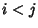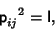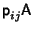## Permutation Matrix

A Matrixobtained by permuting theth andth rows of the Identity Matrix with. Every row and column therefore contain precisely a single 1, and every permutation corresponds to a unique permutation matrix. The matrix is nonsingular, so the Determinant is always Nonzero. It satisfieswhere I is the Identity Matrix. Applying to another Matrix,gives A with theth andth rows interchanged, andgives A with theth andth columns interchanged.

Interpreting the 1s in anpermutation matrix as Rooks gives an allowable configuration of nonattacking Rooks on anChessboard.